Author: Oscar Cronquist Article last updated on May 20, 2022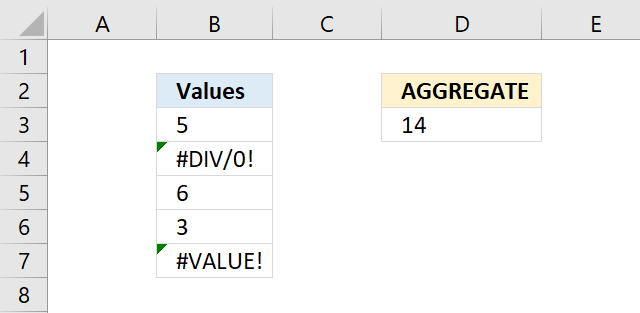The AGGREGATE function allows you to calculate different specific functions to a list or database. A special AGGREGATE function feature is to exclude hidden rows and error values in calculations.

It is designed for columns of data or vertical ranges.

The formula above sums all values in cell range B3:B7 excluding errors.

Formula in cell D3:

=AGGREGATE(9,6,B3:B7)

## 1. AGGREGATE Function Syntax

Reference form

AGGREGATE(function_num, options, ref1, [ref2], …)

Array form

AGGREGATE(function_num, options, array, [k])

## 2. AGGREGATE Function Arguments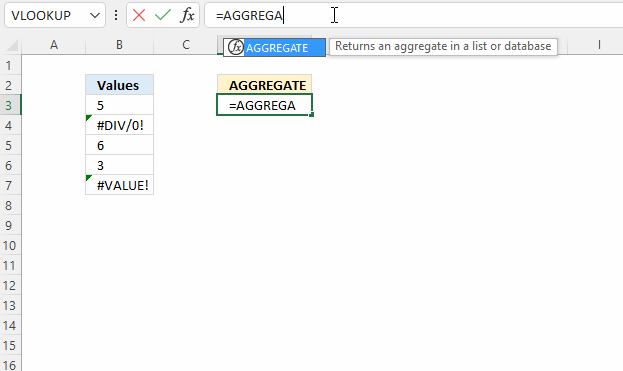The animated image above demonstrates how the AGGREGATE function guides you while selecting the first argument function_num.

 function_num Required. A number 1 to 19 that lets you choose between different functions. See the list below.

 Function 1 AVERAGE 2 COUNT 3 COUNTA 4 MAX 5 MIN. 6 PRODUCT 7 STDEVS.S 8 STDEVS.P 9 SUM 10 VAR.S 11 VAR.P 12 MEDIAN 13 MODE.SNGL 14 LARGE 15 SMALL 16 PERCENTILE.INC 17 QUARTILE.INC 18 PERCENTILE.EXC 19 QUARTILE.EXC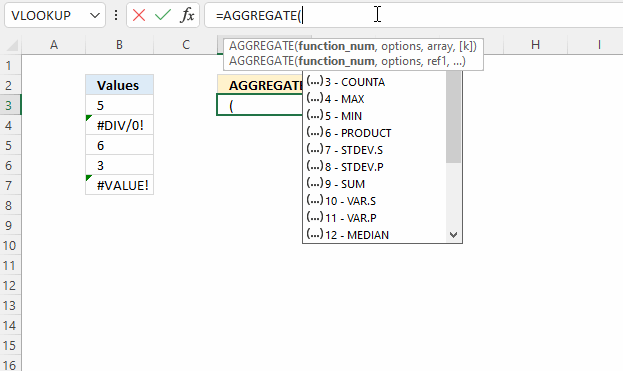The second argument is the options argument, the image above shows what choices you have.

 Options Required. A numerical value that allows you to choose which values to exclude in the calculation.

 0 or omitted. Ignore nested SUBTOTAL and AGGREGATE functions 1 Ignore hidden rows, nested SUBTOTAL and AGGREGATE functions 2 Ignore error values, nested SUBTOTAL and AGGREGATE functions 3 Ignore hidden rows, error values, nested SUBTOTAL and AGGREGATE functions 4 Ignore nothing 5 Ignore hidden rows 6 Ignore error values 7 Ignore hidden rows and error values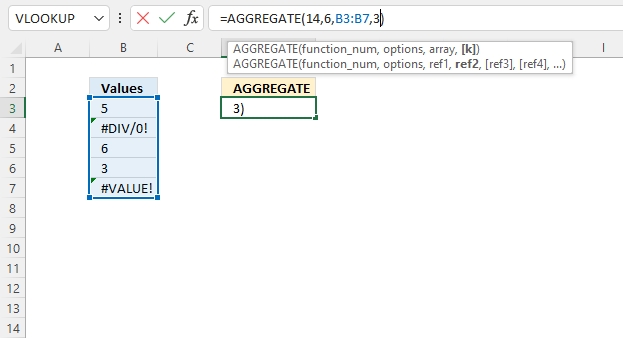The AGGREGATE function has two different forms: array and reference. The array form allows you to use the following functions:

• LARGE(array,k)
• SMALL(array,k)
• PERCENTILE.INC(array,k)
• QUARTILE.INC(array,quart)
• PERCENTILE.EXC(array,k)
• QUARTILE.EXC(array,quart)

The reference form has these arguments:

AGGREGATE(function_num, options, ref1, [ref2], …)

 Ref1 Required. The first numeric argument for functions that take multiple numeric arguments for which you want the aggregate value. Ref2,... Optional. Numeric arguments 2 to 253 for which you want the aggregate value.

How do you choose the AGGREGATE form?
It depends on what function_num number you pick.

## 3. AGGREGATE function examples

### 3.1 AGGREGATE function - calculate the average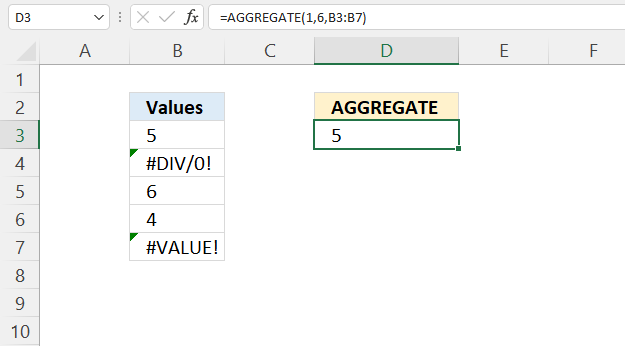The AVERAGE function calculates an average, however, it doesn't ignore error values or hidden rows. This is where the AGGREGATE function is useful.

Formula in cell D3:

=AGGREGATE(1, 6, B3:B7)

#### Arguments

function_num - 1    (AVERAGE function)

Array form - AGGREGATE(function_num, options, array, [k])

options - 6                 (Ignore error values)
array - B3:B7
[k] - optional

5 + 6 + 4 equals 15. 15/3 equals 5.

### 3.2 AGGREGATE function - COUNT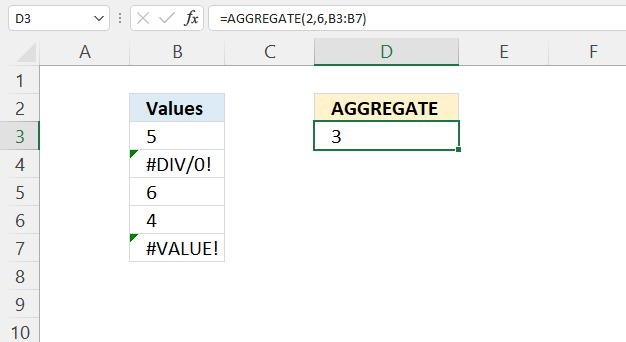Formula in cell D3:

=AGGREGATE(2, 6, B3:B7)

#### Arguments

function_num - 2    (COUNT function)

Array form - AGGREGATE(function_num, options, array, [k])

options - 6                 (Ignore error values)
array - B3:B7
[k] - optional

AGGREGATE(2, 6, B3:B7)

becomes

AGGREGATE(2, 6, {5; #DIV/0!; 6; 4; #VALUE!})

and returns 3. Therer are three numbers in cell range B3:B7

### 3.3 AGGREGATE function - counta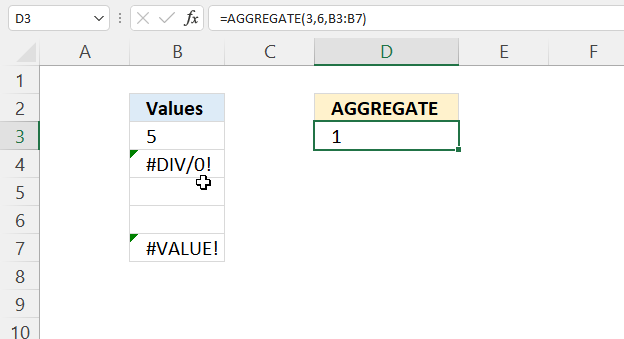Formula in cell D3:

=AGGREGATE(3, 6, B3:B7)

#### Arguments

function_num - 3    (COUNTA function)

Array form - AGGREGATE(function_num, options, array, [k])

options - 6                 (Ignore error values)
array - B3:B7
[k] - optional

AGGREGATE(3, 6, B3:B7)

becomes

AGGREGATE(3, 6, {5; #DIV/0!; ""; ""; #VALUE!})

and returns 1. There is only one non-empty value if you ignore the error values.

### 3.4 AGGREGATE function - max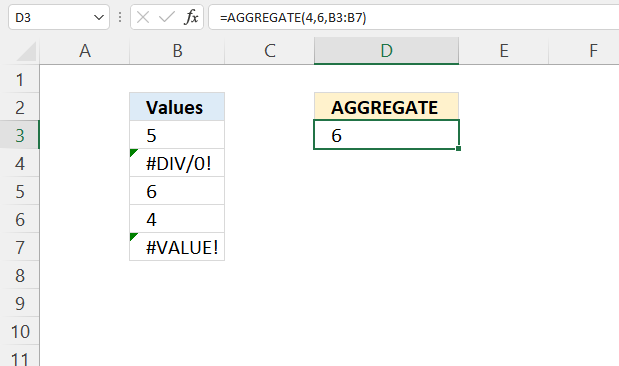Formula in cell D3:

=AGGREGATE(3, 6, B3:B7)

#### Arguments

function_num - 4    (MAX function)

Array form - AGGREGATE(function_num, options, array, [k])

options - 6                 (Ignore error values)
array - B3:B7
[k] - optional

AGGREGATE(3, 6, B3:B7)

becomes

AGGREGATE(4, 6, {5; #DIV/0!; 6; 4; #VALUE!})

and returns 6. Six is the largest number in cell range B3:B7.

### 3.5 AGGREGATE function - min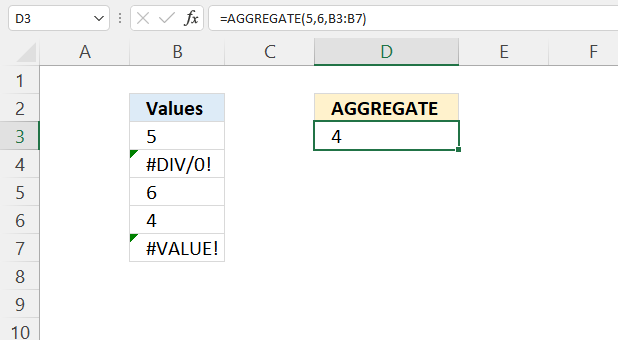Formula in cell D3:

=AGGREGATE(5, 6, B3:B7)

#### Arguments

function_num - 5    (MIN function)

Array form - AGGREGATE(function_num, options, array, [k])

options - 6                 (Ignore error values)
array - B3:B7
[k] - optional

AGGREGATE(5, 6, B3:B7)

becomes

AGGREGATE(5, 6, {5; #DIV/0!; 6; 4; #VALUE!})

and returns 4. Four is the smallest number in cell range B3:B7.

### 3.6 AGGREGATE function - product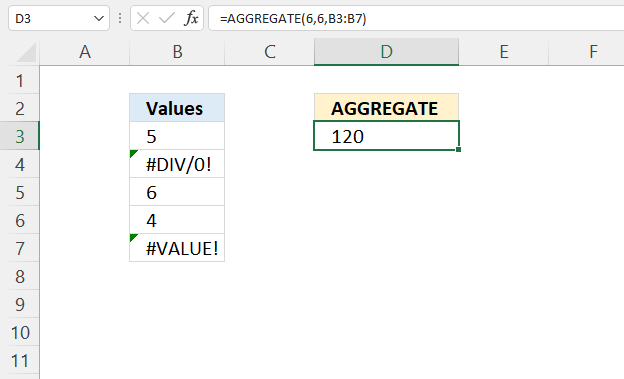Formula in cell D3:

=AGGREGATE(6, 6, B3:B7)

#### Arguments

function_num - 6    (PRODUCT function)

Array form - AGGREGATE(function_num, options, array, [k])

options - 6                 (Ignore error values)
array - B3:B7
[k] - optional

AGGREGATE(6, 6, B3:B7)

becomes

AGGREGATE(6, 6, {5; #DIV/0!; 6; 4; #VALUE!})

and returns 120. 5 * 6 * 4 equals 120.

### 3.7 AGGREGATE function - sum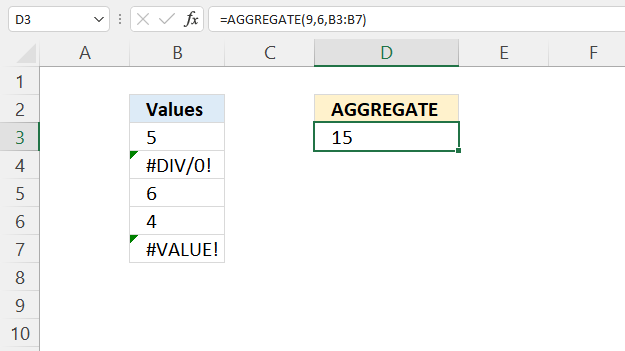Formula in cell D3:

=AGGREGATE(9, 6, B3:B7)

#### Arguments

function_num - 9    (SUM function)

Array form - AGGREGATE(function_num, options, array, [k])

options - 6                 (Ignore error values)
array - B3:B7
[k] - optional

AGGREGATE(6, 6, B3:B7)

becomes

AGGREGATE(6, 6, {5; #DIV/0!; 6; 4; #VALUE!})

and returns 120. 5 * 6 * 4 equals 120.

## 4. What will the AGGREGATE function not exclude?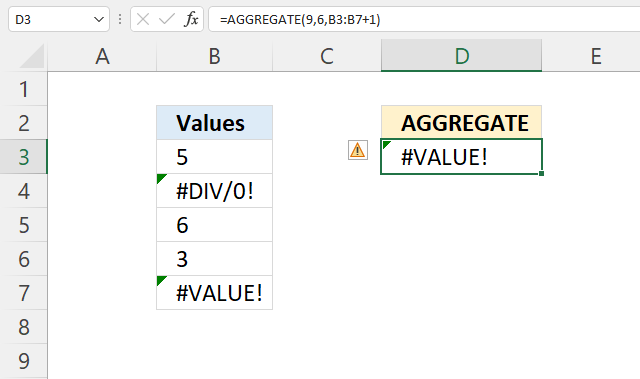The function will not exclude

• hidden rows
• nested subtotals
• nested aggregates if the array argument includes a calculation, for example:
=AGGREGATE(14,3,A1:A10*(A1:A10<1),1)

When you type the first argument in the AGGREGATE function Excel returns a list of functions that are valid.

## 5. How to extract the largest number ignoring error values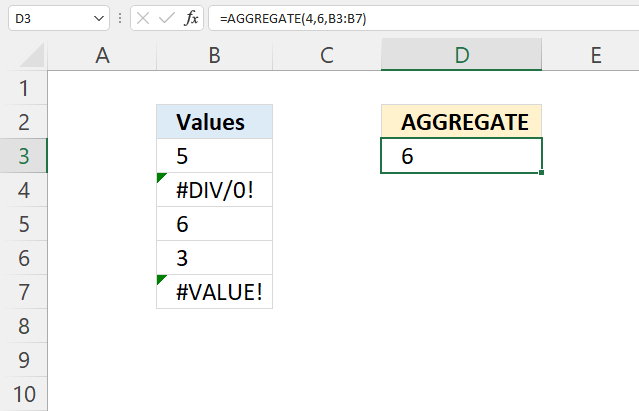The image above demonstrates how the AGGREGATE function is able to return the largest number and at the same time ignore error values.

Formula in cell D3:

=AGGREGATE(4, 6, B3:B7)

This works fine as long as you are using cell references, however, it won't work if you try to use other functions in the third argument or use array values.